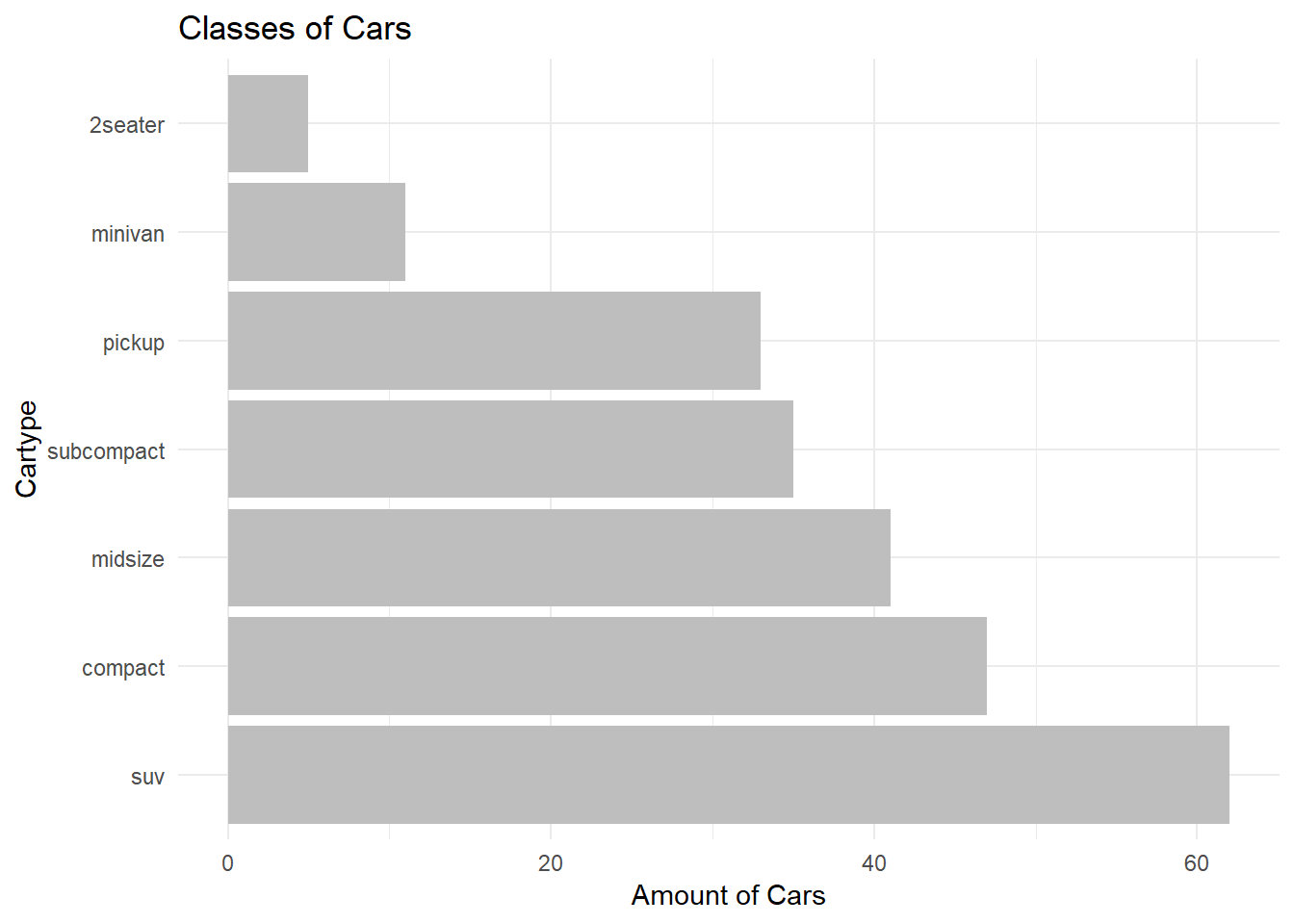I believe learning GGPlot is a good start for everyone who is not familiar with R, but who has at least some coding experience from other statistic programs. For this reason, I truly believe that learning GGPlot is smooth way to learn R ;)

GGPlot is a powerful library that comes with many different applications to visualize data. It starts with very simple things like bar charts, box plots and line charts. Being more familiar with R you can use GGplot even to make maps, animations and other fancy things. We will cover that later, let’s start with some simple graphs to get you an idea how the GG universe works.

As always, we need to install the package install.packages("ggplot2") first and load the library library(ggplot2). Plus, we load two datasets included in R. So you can simply copy the code, fool around and in the end adjust for our own purposes.

#install.packages("XX") to install a package
library(ggplot2)
data(diamonds, mpg, package = "ggplot2")

## Pure and simple bar charts

In the first step we produce a bar chart and adjust it step by step. Let’s say you want to visualize the outcome between two or more groups. So we can start to create a data frame in R with a variable for a group (here a treatment and control group) and some random values.

dat <- data.frame(
Group = factor(c("Treatment","Control"), levels=c("Treatment","Control")),
Outcome = c(18.89, 14.23)
)

Plotting these values is pretty easy. We create a ggplot by defining our dataset (data=dat) and tell ggplot which are our variables of interest and the way to display it.

# Very basic bar graph
ggplot(data=dat, aes(x=Group, y=Outcome)) +
geom_bar(stat="identity")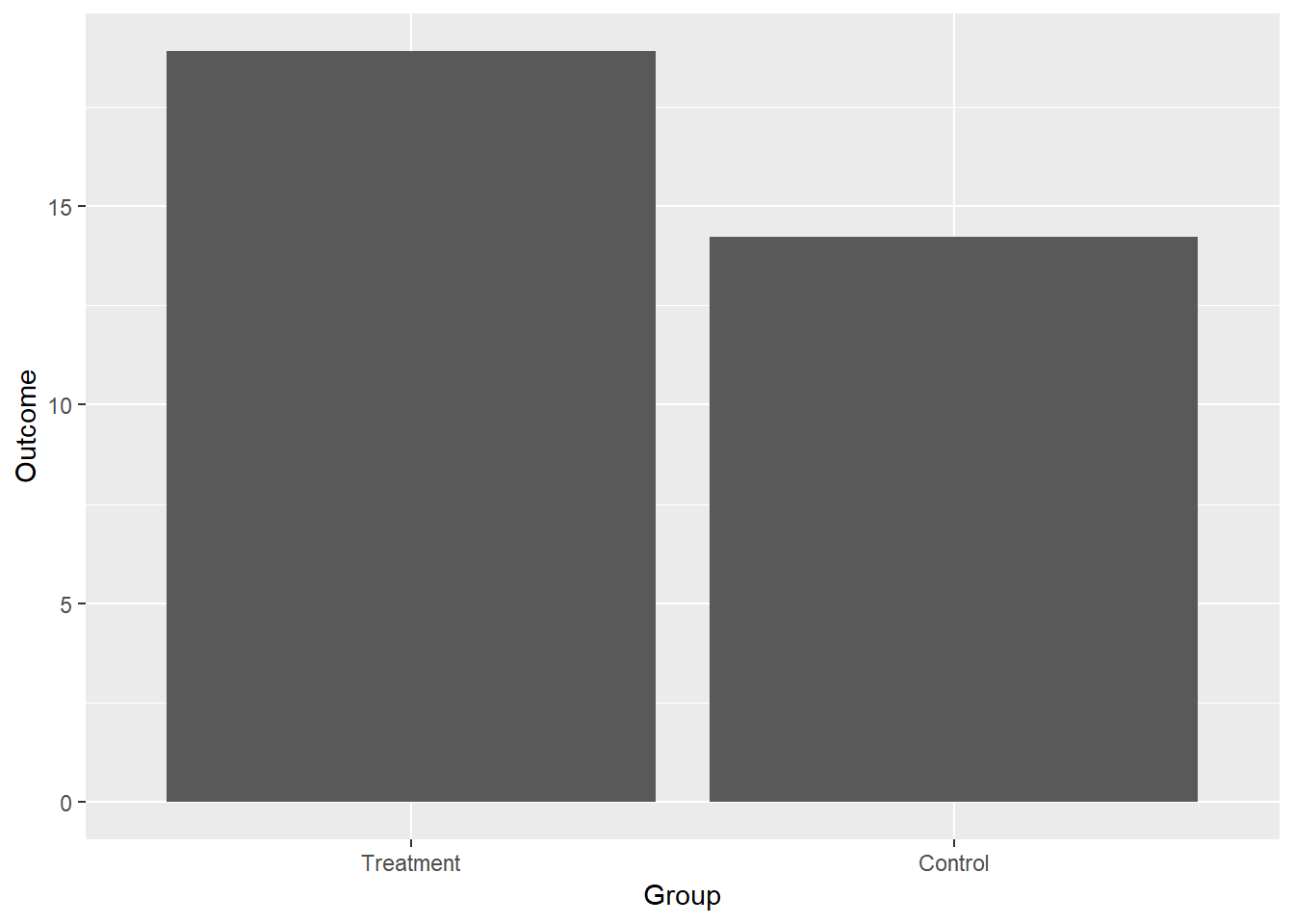So, the basic command displays the numbers for each group since we provided a data frame with the final values. We add the layer geom_bar(stat="identity") to the GGplot to call these values which leaves the y value unchanged. If you instead use geom_bar(stat_bin) than R counts the cases, and we can even use a numerical indicator and specify a group variable group=xx to display the values for each group.

So, this is how the basic syntax works. From here on we can adjust the plot for our purposes (and audience) by adding (+) layers.

## Make it look nice and publishable

Let’s use the basic chart to learn a few options for ggplot while producing a chart you like to include in your own work without being embarrassed :) First we can use diffrent colours to indicate the differences between the groups. Ggplot automatically provides different colours by adding a third variable or instead say fill=Group to our basic command.

# Very basic bar graph
ggplot(data=dat, aes(x=Group, y=Outcome, fill=Group)) +
geom_bar(stat="identity")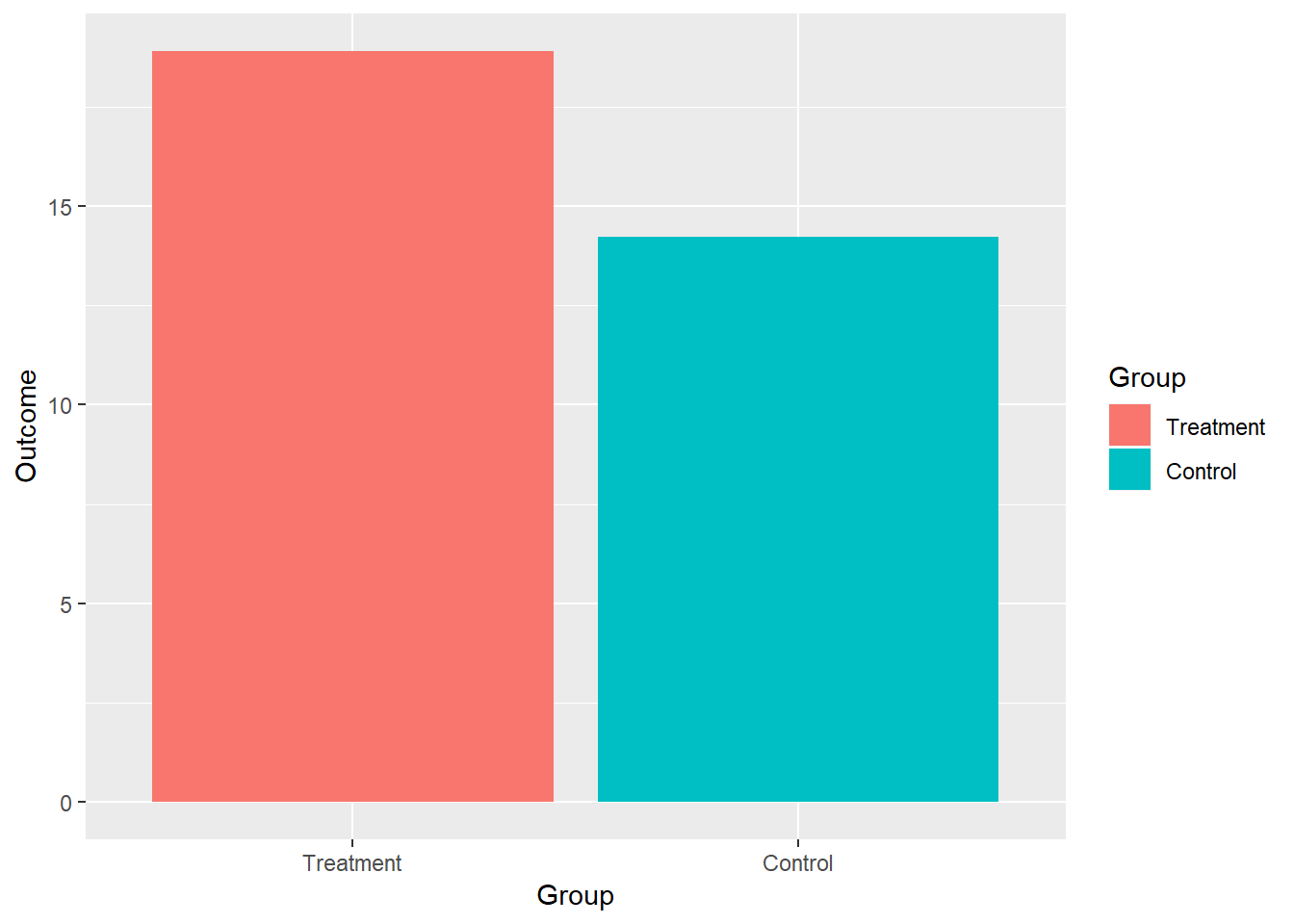You don’t like the colours? Never mind. You can and should adjust them. For example, you can provide a graph with nice colours to highlight the differences in a presentation, but may change the colours to black and white your work. You can work that out with one line of code.

# Very basic bar graph
ggplot(data=dat, aes(x=Group, y=Outcome, fill=Group)) +
geom_bar(stat="identity")+
scale_fill_manual(values=c("black", "gray"))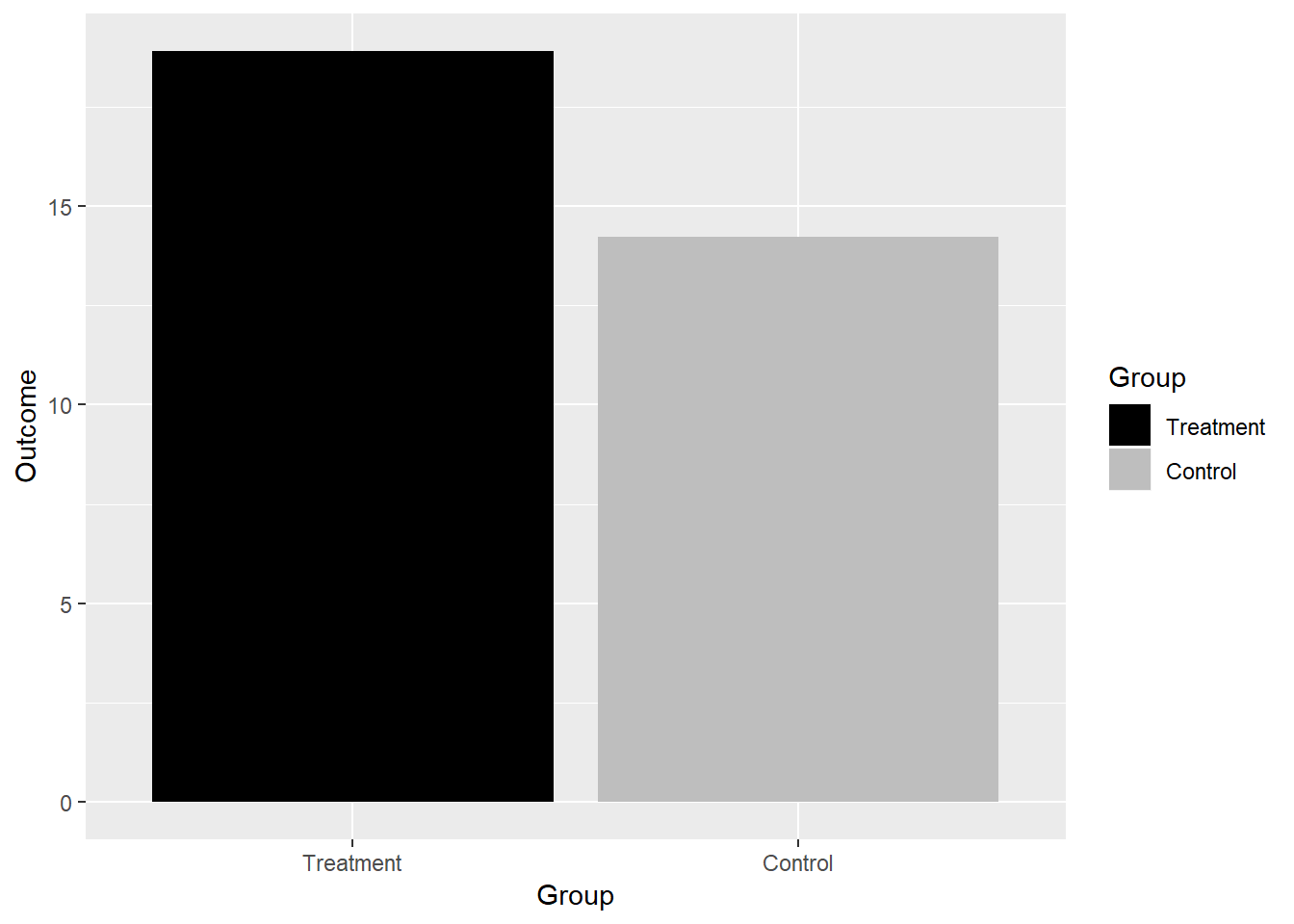Note that we tell ggplot “values” for the colours. This implies that R knows some standard colours, but you can provide R with the hexadecimal format for different colours, which makes it possible to use every colour. Sounds complicated in the first place, but it isn’t. Go to Colorbrewer pick some colours you like, copy the hex code instead of providing the label for the colour.

I go with some blues :).

# Very basic bar graph
ggplot(data=dat, aes(x=Group, y=Outcome, fill=Group)) +
geom_bar(stat="identity")+
scale_fill_manual(values=c("#08519c", "#6baed6"))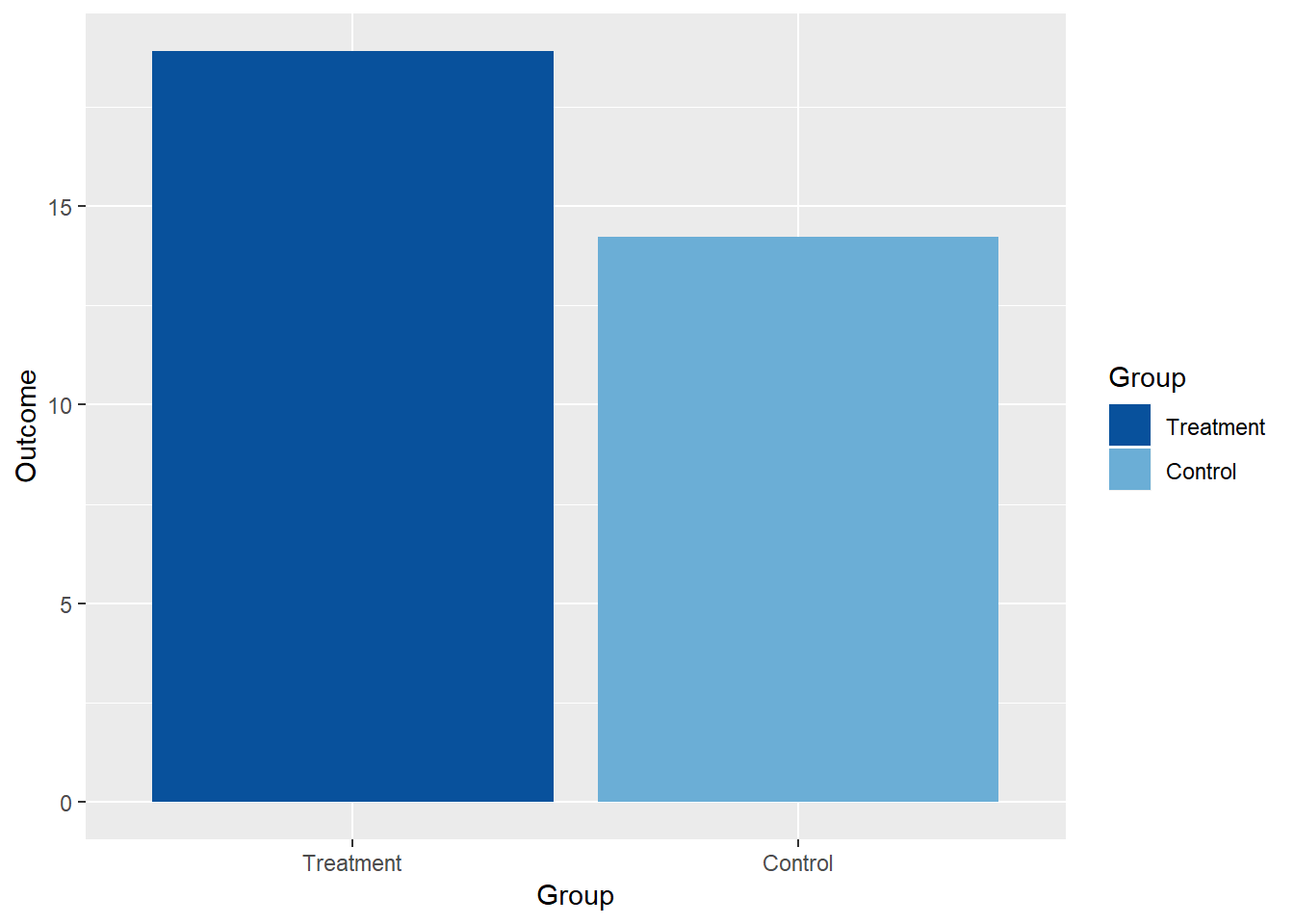To be honest, there is a reason why I tell you this stuff. You can find patterns of nice colours for the nature of your data (sequential, diverging) on colorbrewer, you can adjust your colours to provide colorblind safe, print friendly and photocopy safe graphs. So there are many good reasons why you should think about colours. And that’s especially true if you think about the message you like to transport with your graph. Maybe this is something to decide before we can call it a day. However, if you don’t want to choose different colours use simply scale_colour_brewer() to provide different already defined colour patterns (palettes). Let’s have a look.

# Very basic bar graph
ggplot(data=dat, aes(x=Group, y=Outcome, fill=Group)) +
geom_bar(stat="identity")+
scale_fill_brewer(palette="Set1")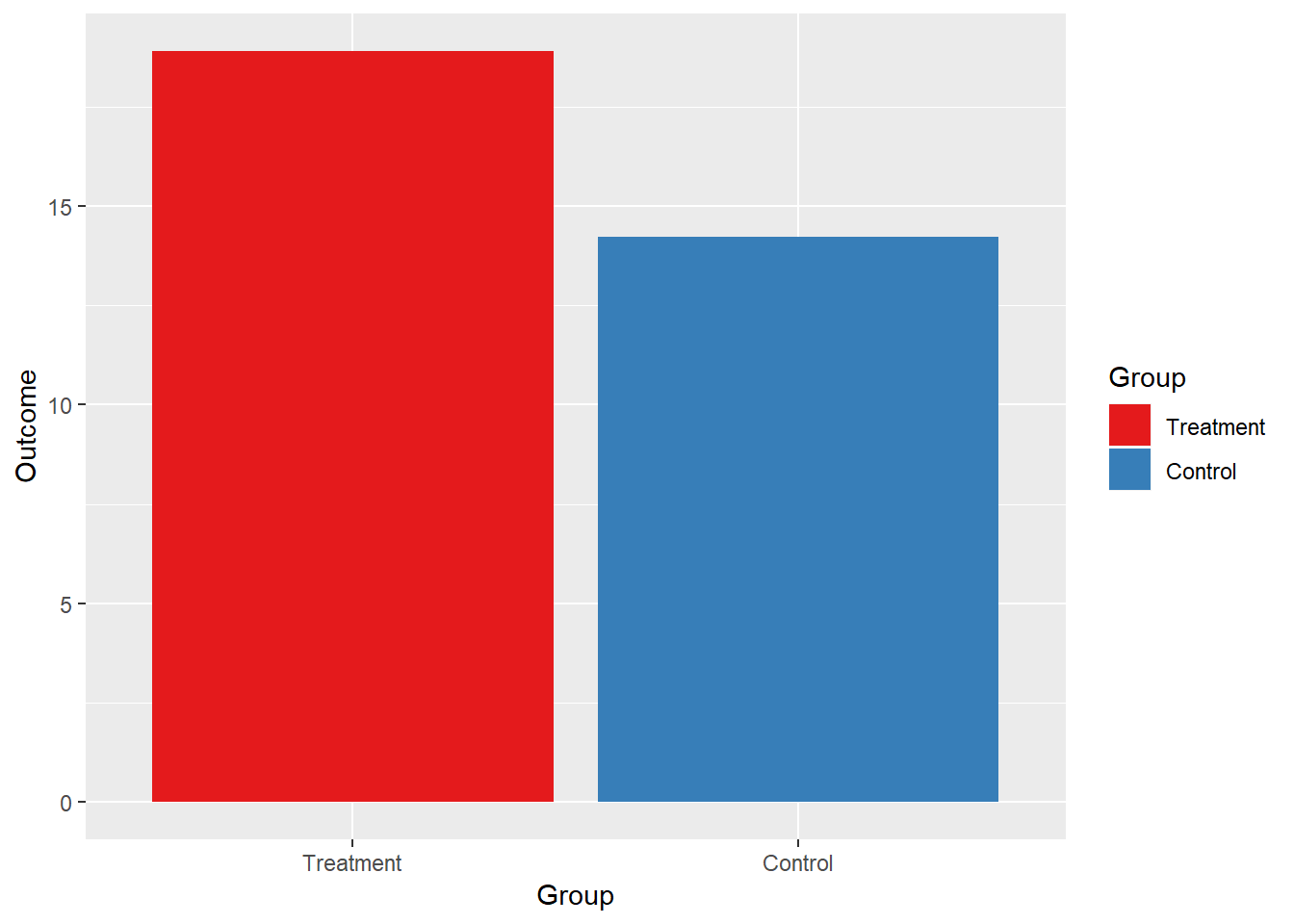Have a look at different defined colour patterns (Set 1,2,3; Reds, Greens), google is our friend. And the same applies for themes to define the background colours, type, size and so one. Certainly, you can define every detail as you wish, but ggplot come with nice themes you can try as a start. Just write theme in a newline and see which suggestions you get for different themes. Here I add the minimalist theme, but you can try different themes (theme_bw(),theme_economist()) and adjust them if necessary.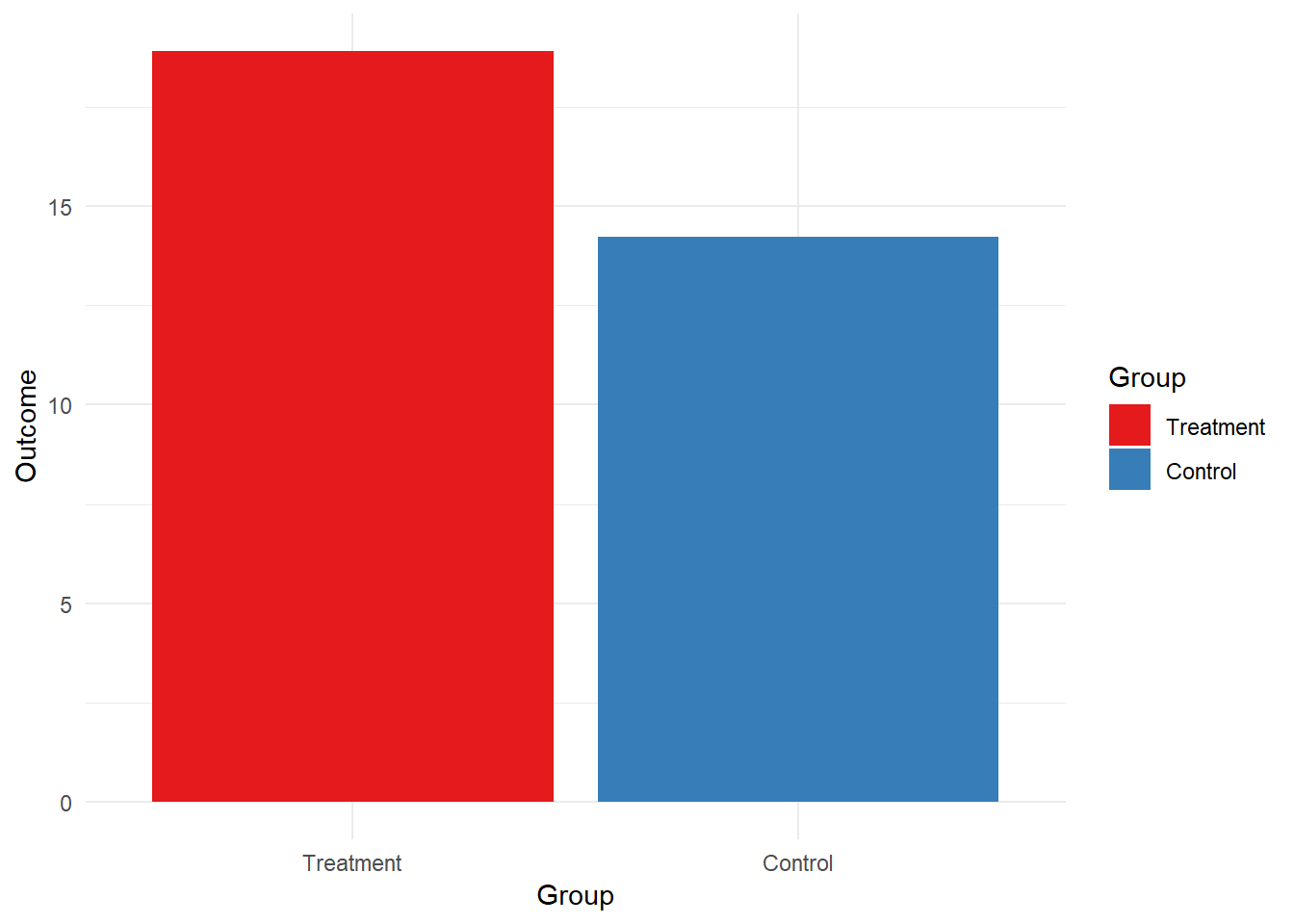Your data is probably not as clean and nice as our small world example. Maybe the variables in the data set do not even have a proper name or label. We should give our audience provide the information that is necessary to understand the graph.

Labels and proper information is not a problem: Just give ggplot labels for the y and or x axis ylab("Label").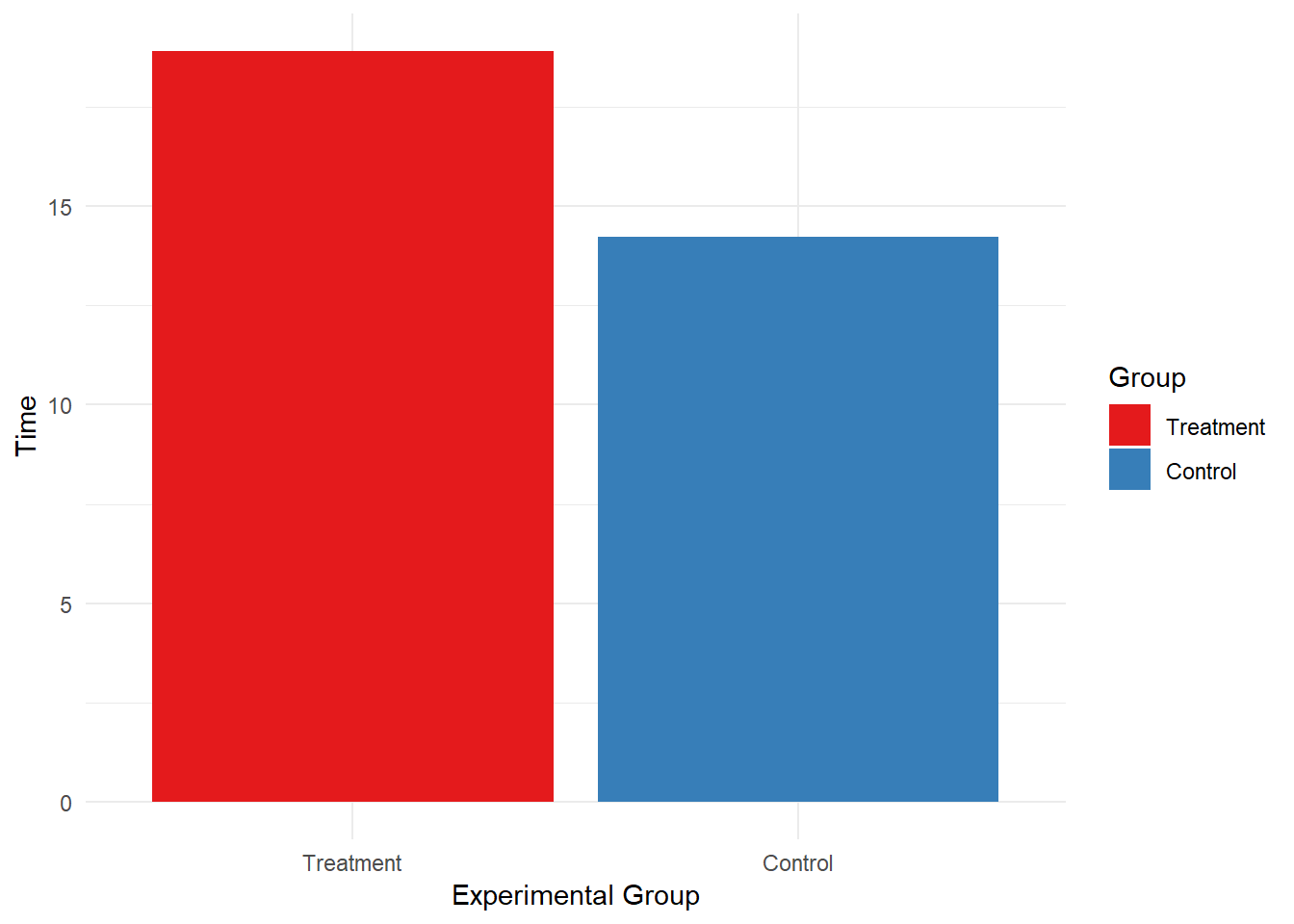# It’s up to you …

There are many different options to make your graph more accessible. Certainly, I don’t believe we need to discuss all options in detail but I provide you some examples (and comments after the # in the code) for each option.

So it’s up to you which information and style to add in your first ggplot. Just give it a try:

• #Moves (left,right, top) and Removes the legend ("none"):
• theme(legend.position="bottom")
• #If your want ajust the order:
• scale_fill_discrete(breaks=c("Control","Treatment"))
• #You can adjust the y (and x) scale by providing the min and max values:
• coord_cartesian(ylim = c(0, 40))
• #You can provide a reference line for the y (and x) variable
• geom_hline(aes(yintercept=20), colour="#990000", linetype="dashed")
• #Provide a title for your graph
• ggtitle("My first ggplot")
• #Change the orientation of the graph: Vertical vs. horizontal
• coord_flip()

Well then, let’s practice a bit. We can use the build-in dataset mpg to make a ranked bar chart. Let’s see how the data looks:

#The mpg dataset
head(mpg)
## # A tibble: 6 x 11
##   manufacturer model displ  year   cyl trans  drv     cty   hwy fl    class
##   <chr>        <chr> <dbl> <int> <int> <chr>  <chr> <int> <int> <chr> <chr>
## 1 audi         a4      1.8  1999     4 auto(~ f        18    29 p     comp~
## 2 audi         a4      1.8  1999     4 manua~ f        21    29 p     comp~
## 3 audi         a4      2    2008     4 manua~ f        20    31 p     comp~
## 4 audi         a4      2    2008     4 auto(~ f        21    30 p     comp~
## 5 audi         a4      2.8  1999     6 auto(~ f        16    26 p     comp~
## 6 audi         a4      2.8  1999     6 manua~ f        18    26 p     comp~

Can you display how many classes of cars are in the mpg dataset? Hint: geom_bar(stat="count")